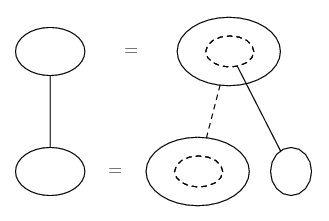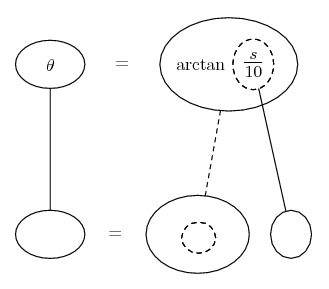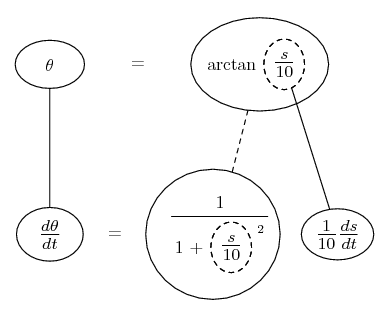# Thread: rate question

1. ## rate question

A prisoner walks along a straight path at a rate of 1.5 meters per second. a spot light is on the ground 10 meters from the path and rotates to keep shining on the prisoner. At what rate is the light rotating when the man is 20 meters from the point on the path closes to the light and is walking away from that point?

2.Originally Posted by haebinparkA prisoner walks along a straight path at a rate of 1.5 meters per second. a spot light is on the ground 10 meters from the path and rotates to keep shining on the prisoner. At what rate is the light rotating when the man is 20 meters from the point on the path closes to the light and is walking away from that point?
The way that this is worded doesn't make any sense. Could you describe the situation a little better, please.

3. If there's a t missing off the end of 'closes' then we have a right triangle with the adjacent side 10 and opposite side currently 20.

Related rates nearly always depend on the chain rule, so you might want to try filling up this pattern...... where straight continuous lines differentiate downwards (integrate up) with respect to the main variable (in this case time), and the straight dashed line similarly but with respect to the dashed balloon expression (the inner function of the composite which is subject to the chain rule).

So what have we got here? theta the angle, s the opposite side of the right triangle, 10 the length of the adjacent, ds/dt the rate of increase of s, and arctan getting theta from s and 10, as here...So differentiate with respect to the inner function, and the inner function with respect to t (I'll put this in a spoiler if I can figure out how)...

Spoiler:... and sub in the given values of s and ds/dt

__________________________________________

Don't integrate - balloontegrate!

Balloon Calculus: Gallery

Balloon Calculus Drawing with LaTeX and Asymptote!

#### Search Tags

question, rate